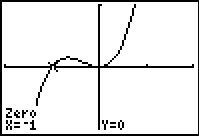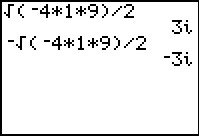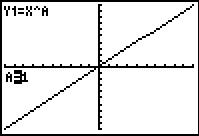# Activities

••• ##### Subject Area

• Math: Precalculus: Polynomial, Power, and Rational Functions

• ##### Author9-12

40 Minutes

• ##### Device
• TI-83 Plus Family
• TI-84 Plus
• TI-84 Plus Silver Edition
•TI-84 Plus C Silver Edition
•TI-84 Plus CE
• ##### Software

TI Connect™
TI Connect™ CE

• ##### Report an Issue

Going Back To Your Roots#### Activity Overview

Students apply the Fundamental Theorem of Algebra in determining the complex roots of polynomial functions.

#### Key Steps

•In this activity, students will explore the application of the Fundamental Theorem of Algebra in determining the number of complex roots of polynomial functions. They will graph a function to find the roots and then see how the multiplicity of the roots compares to the degree of the polynomial.

•Students will determine how many complex roots and what type of roots the function has by graphing. Then they will determine the value of imaginary roots. Students will also investigate a polynomial that has both real and imaginary roots.

•The effect of multiplicity of roots on the graph of a function is further explored via the Transformational Graphing application.